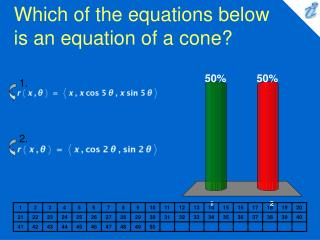# Which of the equations below is an equation of a cone? - PowerPoint PPT PresentationDownload PresentationWhich of the equations below is an equation of a cone?

Which of the equations below is an equation of a cone?
Download Presentation## Which of the equations below is an equation of a cone?

- - - - - - - - - - - - - - - - - - - - - - - - - - - E N D - - - - - - - - - - - - - - - - - - - - - - - - - - -
##### Presentation Transcript

1. 1. 2. Which of the equations below is an equation of a cone? • {image} • {image}

2. 1. 2. Which of the given equations is the equation of the following surface? {image} • {image} • {image}

3. 1. 2. 3. 4. Find a parametric representation for the part of the cylinder {image} that lies between the planes y = -9 and y = 4. • {image} • {image} • {image} • {image}

4. 1. 2. 3. 4. Find a parametric representation for the part of the plane z = x + 8 that lies inside the cylinder {image} . • {image} • {image} • {image} • {image}

5. 1. 2. 3. 4. 5. Find the area of the surface with parametric equations x = uv, y = u + v, z = u - v, {image} • {image} • {image} • {image} • {image} • {image}